cwtfilterbank

Continuous wavelet transform filter bank

Description

Use cwtfilterbank to create a continuous wavelet transform (CWT) filter bank. The default wavelet used in the filter bank is the analytic Morse (3,60) wavelet. You can vary the time-bandwidth and symmetry parameters for the Morse wavelets, to tune the Morse wavelet for your needs. You can also use the analytic Morlet (Gabor) wavelet or bump wavelet. When analyzing multiple signals in time-frequency, for improved computational efficiency, you can precompute the filters once and then pass the filter bank as input to cwt. With the filter bank, you can visualize wavelets in time and frequency. You can also create filter banks with specific frequency or period ranges, and measure 3-dB bandwidths. You can determine the quality factor for the wavelets in the filter bank.

Creation

Description

example

fb = cwtfilterbank creates a continuous wavelet transform (CWT) filter bank fb. The filters are normalized so that the peak magnitudes for all passbands are approximately equal to 2. The default filter bank is designed for a signal with 1024 samples. The default filter bank uses the analytic Morse (3,60) wavelet. The filter bank uses the default scales: approximately 10 wavelet bandpass filters per octave (10 voices per octave). The highest-frequency passband is designed so that the magnitude falls to half the peak value at the Nyquist frequency.

As implemented, the CWT uses L1 normalization. With L1 normalization, equal amplitude oscillatory components at different scales have equal magnitude in the CWT. L1 normalization provides a more accurate representation of the signal. The amplitudes of the oscillatory components agree with the amplitudes of the corresponding wavelet coefficients. See Sinusoid and Wavelet Coefficient Amplitudes.

fb can be used as input for cwt.

example

fb = cwtfilterbank(Name,Value) creates a CWT filter bank fb with properties specified by one or more Name,Value pair arguments. Properties can be specified in any order as Name1,Value1,...,NameN,ValueN. Enclose each property name in quotes.

Note

You cannot change a property value of an existing filter bank. For example, if you have a filter bank fb with a SignalLength of 2000, you must create a second filter bank fb2 to process a signal with 2001 samples. You cannot assign a different SignalLength to fb.

Properties

expand all

Length of the signal, specified as a positive integer. The signal must have at least four samples.

Example: 'SignalLength',1700

Data Types: double

Analysis wavelet used in the filter bank, specified as 'Morse', 'amor', or 'bump'. These strings specify the analytic Morse, Morlet (Gabor), and bump wavelet, respectively. The default wavelet is the analytic Morse (3,60) wavelet.

By default, for Morse wavelets, the frequency response decays to 50% of the peak magnitude at the Nyquist. For the Morlet and bump wavelets, the frequency response decays to 10% of the peak magnitude. You can change the decay percentage by setting the filter bank FrequencyLimits property. See cwtfreqbounds.

For Morse wavelets, you can also parameterize the wavelet using the TimeBandwidth or WaveletParameters properties.

Example: 'Wavelet','bump'

Number of voices per octave to use for the CWT, specified as an even integer from 4 to 48. The CWT scales are discretized using the specified number of voices per octave. The energy spread of the wavelet in frequency and time automatically determines the minimum and maximum scales.

You can use cwtfreqbounds to determine the frequency limits of the wavelet filter bank. The frequency limits depend on parameters such as the energy spread of the wavelet, number of voices per octave, signal length, and sampling frequency.

Example: 'VoicesPerOctave',20

Data Types: single | double

Sampling frequency in hertz, specified as a positive scalar. If unspecified, frequencies are in cycles/sample and the Nyquist frequency is ½. To specify scales in periods, use the SamplingPeriod and PeriodLimits properties.

You cannot specify both the SamplingFrequency and SamplingPeriod properties.

Example: 'SamplingFrequency',5

Data Types: single | double

Frequency limits of the wavelet filter bank, specified as a two-element vector with positive strictly increasing entries. The first element specifies the lowest peak passband frequency. The frequency must be greater than or equal to the product of the wavelet peak frequency in hertz and two time standard deviations divided by the signal length. The high frequency limit must be less than or equal to the Nyquist. The base-2 logarithm of the ratio of the high frequency limit, fMax, to the low frequency limit, fMin, must be greater than or equal to 1/NV, where NV is the number of voices per octave:

log2(fMax/fMin) ≥ 1/NV.

If you specify frequency limits outside the permissible range, cwtfilterbank truncates the limits to the minimum and maximum values. Use cwtfreqbounds to determine frequency limits for different parametrizations of the wavelet transform.

If using a sampling period in the filter bank, you cannot specify the FrequencyLimits property.

Example: If fb = cwtfilterbank('SignalLength',1000,'SamplingFrequency',1000,'FrequencyLimits',[90 100]), then log2(100/90) ≥ 1/fb.VoicesPerOctave.

Data Types: double

Sampling period, specified as a scalar duration. You cannot specify both the SamplingFrequency and SamplingPeriod properties.

Example: 'SamplingPeriod',seconds(0.5)

Data Types: duration

Period limits of the wavelet filter bank, specified as a two-element duration array with positive strictly increasing entries. The first element of PeriodLimits specifies the largest peak passband frequency and must be greater than or equal to twice the SamplingPeriod. The maximum period cannot exceed the signal length divided by the product of two time standard deviations of the wavelet and the wavelet peak frequency. The base-2 logarithm of the ratio of the minimum period, minP, to the maximum period, maxP, must be less than or equal to -1/NV, where NV is the number of voices per octave:

log2(minP/maxP) ≤ -1/NV.

If you specify period limits outside the permissible range, cwtfilterbank truncates the limits to the minimum and maximum values. Use cwtfreqbounds to determine period limits for different parametrizations of the wavelet transform.

If using a sampling frequency in the filter bank, you cannot specify the PeriodLimits property.

Example: If fb = cwtfilterbank('SignalLength',1000,'SamplingPeriod',seconds(0.1),'PeriodLimits',[seconds(0.2) seconds(3)]), then log2(0.2/3) ≤ -1/fb.VoicesPerOctave.

Data Types: duration

Time-bandwidth product for Morse wavelets, specified as a positive scalar. This property is only valid when the Wavelet property is 'morse'. This property specifies the time-bandwidth product of the Morse wavelet with the symmetry parameter (gamma) fixed at 3. TimeBandwidth is a positive number strictly greater than 3 and less than or equal to 120.

The larger the time-bandwidth product, the more spread out the wavelet is in time and narrower the wavelet is in frequency. The standard deviation of the Morse wavelet in time is approximately sqrt(TimeBandwidth/2). The standard deviation in frequency is approximately 1/2*sqrt(2/TimeBandwidth). See Generalized Morse and Analytic Morlet Wavelets.

The TimeBandwidth and WaveletParameters properties cannot both be specified.

In the notation of Morse Wavelets, TimeBandwidth is P2.

Example: 'TimeBandwidth',20

Data Types: double

Morse wavelet parameters, specified as a two-element vector. The first element is the symmetry parameter (gamma), which must be greater than or equal to 1. The second element is the time-bandwidth product, which must be strictly greater than gamma. The ratio of the time-bandwidth product to gamma cannot exceed 40.

When gamma is equal to 3, the Morse wavelet is perfectly symmetric in the frequency domain. The skewness is equal to 0. Values of gamma greater than 3 result in positive skewness, while values of gamma less than 3 result in negative skewness. WaveletParameters is only valid if the Wavelet property is set to 'Morse'.

The WaveletParameters and TimeBandwidth properties cannot both be specified.

Example: 'WaveletParameters',[4,20]

Boundary extension of signal, specified as either 'reflection' or 'periodic'. Determines how the data is treated at the boundary.

Example: 'Boundary','periodic'

Object Functions

 wt Continuous wavelet transform with filter bank freqz CWT filter bank frequency responses timeSpectrum Time-averaged wavelet spectrum scaleSpectrum Scale-averaged wavelet spectrum wavelets CWT filter bank time-domain wavelets scales CWT filter bank scales waveletsupport CWT filter bank time supports qfactor CWT filter bank quality factor powerbw CWT filter bank 3 dB bandwidths centerFrequencies CWT filter bank bandpass center frequencies centerPeriods CWT filter bank bandpass center periods

Examples

collapse all

Create a continuous wavelet transform filter bank.

fb = cwtfilterbank
fb =
cwtfilterbank with properties:

VoicesPerOctave: 10
Wavelet: 'Morse'
SamplingFrequency: 1
SamplingPeriod: []
PeriodLimits: []
SignalLength: 1024
FrequencyLimits: []
TimeBandwidth: 60
WaveletParameters: []
Boundary: 'reflection'

Plot the magnitude frequency response.

freqz(fb)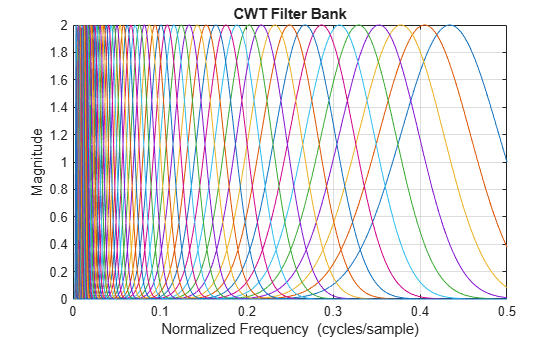Create two sine waves with frequencies of 16 and 64 Hz. The data is sampled at 1000 Hz. Plot the signal.

Fs = 1e3;
t = 0:1/Fs:1-1/Fs;
x = cos(2*pi*64*t).*(t>=0.1 & t<0.3)+sin(2*pi*16*t).*(t>=0.5 & t<0.9);
plot(t,x)
title('Signal')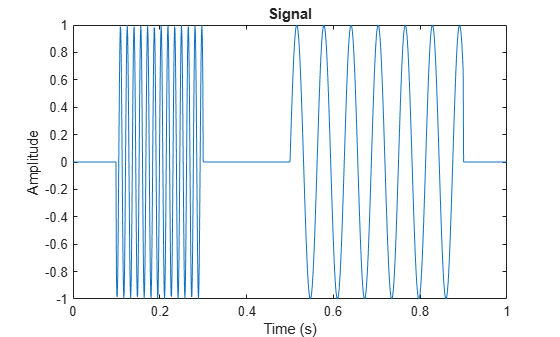Create a CWT filter bank for the signal. Plot the frequency responses of the wavelets in the filter bank.

fb = cwtfilterbank('SignalLength',numel(t),'SamplingFrequency',Fs);
freqz(fb)
title('Frequency Responses — Morse (3,60) Wavelet')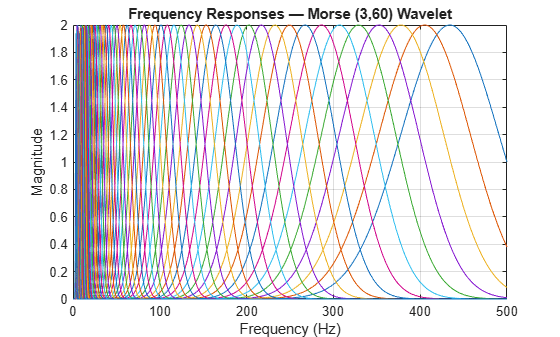The analytic Morse (3,60) wavelet is the default wavelet in the filter bank. The wavelet has a time-bandwidth product equal to 60. Create a second filter bank identical to the first filter bank but instead uses the analytic Morse (3,5) wavelet. Plot the frequency responses of the wavelets in the second filter bank.

fb3x5 = cwtfilterbank('SignalLength',numel(t),'SamplingFrequency',Fs,...
'TimeBandwidth',5);
figure
freqz(fb3x5)
title('Frequency Responses — Morse (3,5) Wavelet')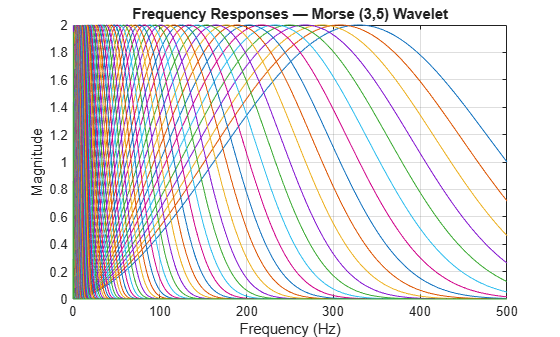Observe that the frequency responses are wider than in the first filter bank. The Morse (3,60) wavelet is better localized in frequency than the Morse (3,5) wavelet. Apply each filter bank to the signal and plot the resulting scalograms. Observe that the Morse (3,60) wavelet has better frequency resolution than the Morse (3,5) wavelet.

figure
cwt(x,'FilterBank',fb)
title('Magnitude Scalogram — Morse (3,60)')figure
cwt(x,'FilterBank',fb3x5)
title('Magnitude Scalogram — Morse (3,5)')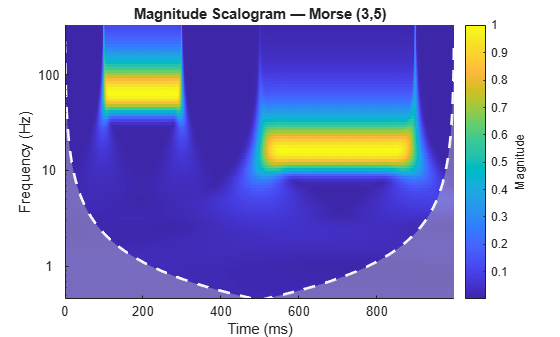This example shows that the amplitudes of oscillatory components in a signal agree with the amplitudes of the corresponding wavelet coefficients.

Create a signal composed of two sinusoids with disjoint support in time. One sinusoid has a frequency of 32 Hz and amplitude equal to 1. The other sinusoid has a frequency of 64 Hz and amplitude equal to 2. The signal is sampled for one second at 1000 Hz. Plot the signal.

frq1 = 32;
amp1 = 1;
frq2 = 64;
amp2 = 2;

Fs = 1e3;
t = 0:1/Fs:1;
x = amp1*sin(2*pi*frq1*t).*(t>=0.1 & t<0.3)+amp2*sin(2*pi*frq2*t).*(t>0.6 & t<0.9);

plot(t,x)
grid on
xlabel('Time (sec)')
ylabel('Amplitude')
title('Signal')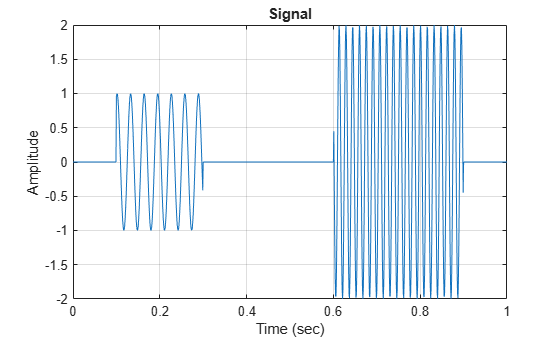Create a CWT filter bank that can be applied to the signal. Since the signal component frequencies are known, set the frequency limits of the filter bank to a narrow range that includes the known frequencies. To confirm the range, plot the magnitude frequency responses for the filter bank.

fb = cwtfilterbank('SignalLength',numel(x),'SamplingFrequency',Fs,...
'FrequencyLimits',[20 100]);
figure
freqz(fb)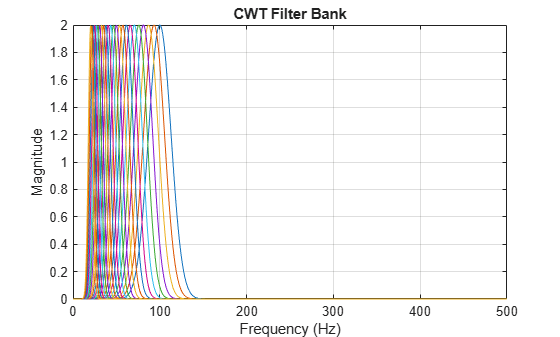Use cwt and the filter bank to plot the scalogram of the signal.

figure
cwt(x,'FilterBank',fb)Execute this script and use a data cursor to confirm that the amplitudes of the wavelet coefficients are essentially equal to the amplitudes of the sinusoidal components.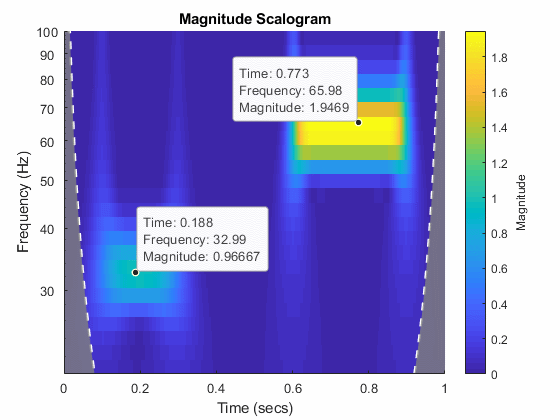This example shows how to vary the time-bandwidth parameter of the generalized Morse wavelet to approximate the analytic Morlet wavelet.

Generalized Morse wavelets are a family of exactly analytic wavelets. Morse wavelets have two parameters, symmetry and time-bandwidth product. You can vary these parameters to obtain analytic wavelets with different properties and behaviors. For additional information, see Morse Wavelets and the references therein.

Load the seismograph data recorded during the 1995 Kobe earthquake. The data are seismograph (vertical acceleration, nm/sq.sec) measurements recorded at Tasmania University, Hobart, Australia on 16 January 1995 beginning at 20:56:51 (GMT) and continuing for 51 minutes at 1 second intervals. Create a CWT filter bank with default settings that can be applied to the data. Use the filter bank to generate the scalogram.

fb = cwtfilterbank('SignalLength',numel(kobe),'SamplingFrequency',1);
cwt(kobe,'FilterBank',fb)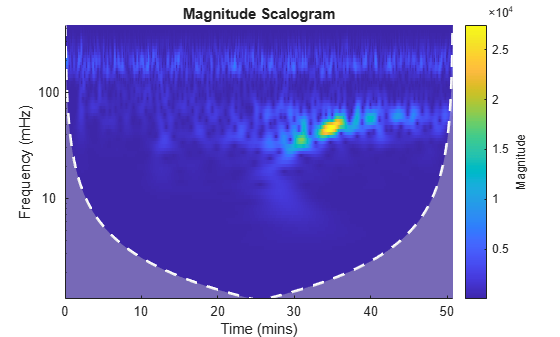The magnitude of the wavelet coefficients is large in the frequency range from 10 mHz to 100 mHz. Create a new filter bank with frequency limits set to these values. Generate the scalogram.

fb2 = cwtfilterbank('SignalLength',numel(kobe),'SamplingFrequency',1,...
'FrequencyLimits',[1e-2 1e-1]);
cwt(kobe,'FilterBank',fb2)
title('Default (3,60) Morse')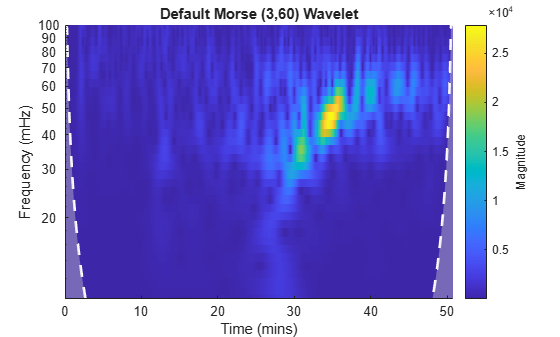By default, cwtfilterbank uses the (3,60) Morse wavelet. Create a filter bank using the analytic Morlet wavelet with the same frequency limits. Generate a scalogram and compare with the scalogram generated by the (3,60) Morse wavelet.

fbMorlet = cwtfilterbank('SignalLength',numel(kobe),'SamplingFrequency',1,...
'FrequencyLimits',[1e-2 1e-1],...
'Wavelet','amor');
cwt(kobe,'FilterBank',fbMorlet)
title('Analytic Morlet')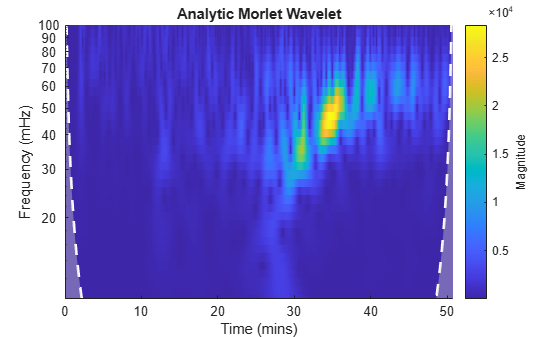The Morlet wavelet is not as well localized in frequency as the (3,60) Morse wavelet. However, by varying the time-bandwidth product, you can create a Morse wavelet with properties similar to the Morlet wavelet.

Create a filter bank using the Morse wavelet with a time-bandwidth value of 30 , with frequency limits as above. Generate the scalogram of the seismograph data. Note there is smearing in frequency nearly identical to the Morlet results.

fbMorse = cwtfilterbank('SignalLength',numel(kobe),'SamplingFrequency',1,...
'FrequencyLimits',[1e-2 1e-1],...
'TimeBandwidth',30);
cwt(kobe,'FilterBank',fbMorse)
title('(3,30) Morse')Now examine the wavelets associated with the fbMorlet and fbMorse filter banks. From both filter banks, obtain the wavelet center frequencies, filter frequency responses, and time-domain wavelets. Confirm the center frequencies are nearly identical.

cfMorlet = centerFrequencies(fbMorlet);
[frMorlet,fMorlet] = freqz(fbMorlet);
[wvMorlet,tMorlet] = wavelets(fbMorlet);
cfMorse = centerFrequencies(fbMorse);
[frMorse,fMorse] = freqz(fbMorse);
[wvMorse,tMorse] = wavelets(fbMorse);

disp(['Number of Center Frequencies: ',num2str(length(cfMorlet))]);
Number of Center Frequencies: 34
disp(['Maximum difference: ',num2str(max(abs(cfMorlet-cfMorse)))]);
Maximum difference: 2.7756e-17

Each filter bank contains the same number of wavelets. Choose a center frequency, and plot the frequency response of the associated filter from each filter bank. Confirm the responses are nearly identical.

wv = 13;
figure
plot(fMorlet,frMorlet(wv,:));
hold on
plot(fMorse,frMorse(wv,:));
grid on
title('Frequency Response')
xlabel('Frequency')
ylabel('Amplitude')
legend('Morlet','(3,30) Morse')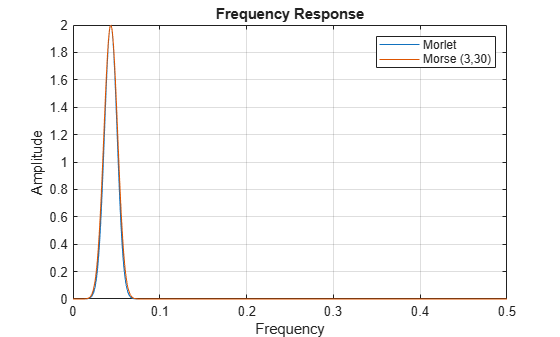Plot the time-domain wavelets associated with the same center frequency. Confirm they are nearly identical.

figure
subplot(2,1,1)
plot(tMorlet,real(wvMorlet(wv,:)))
hold on
plot(tMorse,real(wvMorse(wv,:)))
grid on
title('Real')
legend('Morlet','(3,30) Morse')
xlim([-100 100])
subplot(2,1,2)
plot(tMorlet,imag(wvMorlet(wv,:)))
hold on
plot(tMorse,imag(wvMorse(wv,:)))
grid on
title('Imaginary')
legend('Morlet','(3,30) Morse')
xlim([-100 100])This example shows that increasing the time-bandwidth product ${P}^{2}$ of the Morse wavelet creates a wavelet with more oscillations under its envelope. Increasing ${P}^{2}$ narrows the wavelet in frequency.

Create two filter banks. One filter bank has the default TimeBandwidth value of 60. The second filter bank has a TimeBandwidth value of 10. The SignalLength for both filter banks is 4096 samples.

sigLen = 4096;
fb60 = cwtfilterbank('SignalLength',sigLen);
fb10 = cwtfilterbank('SignalLength',sigLen,'TimeBandwidth',10);

Obtain the time-domain wavelets for the filter banks.

[psi60,t] = wavelets(fb60);
[psi10,~] = wavelets(fb10);

Use the scales function to find the mother wavelet for each filter bank.

sca60 = scales(fb60);
sca10 = scales(fb10);
[~,idx60] = min(abs(sca60-1));
[~,idx10] = min(abs(sca10-1));
m60 = psi60(idx60,:);
m10 = psi10(idx10,:);

Since the time-bandwidth product is larger for the fb60 filter bank, verify the m60 wavelet has more oscillations under its envelope than the m10 wavelet.

subplot(2,1,1)
plot(t,abs(m60))
grid on
hold on
plot(t,real(m60))
plot(t,imag(m60))
xlim([-30 30])
legend('abs(m60)','real(m60)','imag(m60)')
title('TimeBandwidth = 60')
subplot(2,1,2)
plot(t,abs(m10))
grid on
hold on
plot(t,real(m10))
plot(t,imag(m10))
xlim([-30 30])
legend('abs(m10)','real(m10)','imag(m10)')
title('TimeBandwidth = 10')Align the peaks of the m60 and m10 magnitude frequency responses. Verify the frequency response of the m60 wavelet is narrower than the frequency response for the m10 wavelet.

cf60 = centerFrequencies(fb60);
cf10 = centerFrequencies(fb10);

m60cFreq = cf60(idx60);
m10cFreq = cf10(idx10);

freqShift = 2*pi*(m60cFreq-m10cFreq);
x10 = m10.*exp(1j*freqShift*(-sigLen/2:sigLen/2-1));

figure
plot([abs(fft(m60)).' abs(fft(x10)).'])
grid on
legend('Time-bandwidth = 60','Time-bandwidth = 10')
title('Magnitude Frequency Responses')This example shows how using a CWT filter bank improves computational efficiency when taking the CWT of multiple time series.

Load the seismograph data recorded during the 1995 Kobe earthquake. The data are seismograph (vertical acceleration, nm/sq.sec) measurements recorded at Tasmania University, Hobart, Australia on 16 January 1995 beginning at 20:56:51 (GMT) and continuing for 51 minutes at 1 second intervals. Create a CWT filter bank that can be applied to the data.

fb = cwtfilterbank('SignalLength',numel(kobe),'SamplingFrequency',1);

Use the cwt function and take the CWT of the data 250 times. Display the elapsed time used.

num = 250;
tic;
for k=1:num
cfs = cwt(kobe);
end
toc
Elapsed time is 6.551628 seconds.

Now use the wt object function of the filter bank to take the CWT of the data. Confirm using the filter bank is faster.

tic;
for k=1:num
cfs = wt(fb,kobe);
end
toc
Elapsed time is 3.782376 seconds.

This example shows how to plot the CWT scalogram in a figure subplot.

Load the speech sample. The data is sampled at 7418 Hz. Plot the default CWT scalogram.

cwt(mtlb,Fs)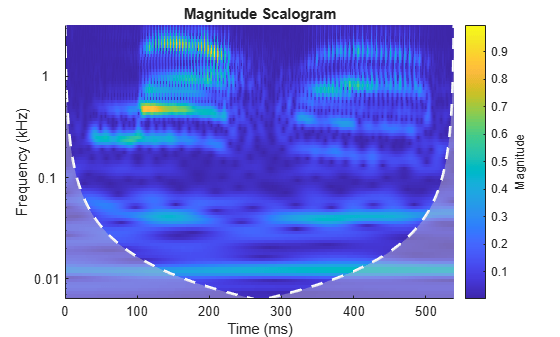Obtain the continuous wavelet transform of the signal, and the frequencies of the CWT.

[cfs,frq] = cwt(mtlb,Fs);

The cwt function sets the time and frequency axes in the scalogram. Create a vector representing the sample times.

tms = (0:numel(mtlb)-1)/Fs;

In a new figure, plot the original signal in the upper subplot and the scalogram in the lower subplot. Plot the frequencies on a logarithmic scale.

figure
subplot(2,1,1)
plot(tms,mtlb)
axis tight
title('Signal and Scalogram')
xlabel('Time (s)')
ylabel('Amplitude')
subplot(2,1,2)
surface(tms,frq,abs(cfs))
axis tight
xlabel('Time (s)')
ylabel('Frequency (Hz)')
set(gca,'yscale','log')Tips

• The first time you use a filter bank to take the CWT of a signal, the wavelet filters are constructed to have the same datatype as the signal. A warning message is generated when you apply the same filter bank to a signal with a different datatype. Changing datatypes comes with the cost of redesigning or changing the precision of the filter bank. For optimal performance, use a consistent datatype.

• When performing multiple CWTs, for example inside a for-loop, the recommended workflow is to first create a cwtfilterbank object and then use the wt object function. This workflow minimizes overhead and maximizes performance. See Using CWT Filter Bank on Multiple Time Series.

Compatibility Considerations

expand all

Not recommended starting in R2018b

 Lilly, J. M., and S. C. Olhede. “Generalized Morse Wavelets as a Superfamily of Analytic Wavelets.” IEEE Transactions on Signal Processing. Vol. 60, No. 11, 2012, pp. 6036–6041.

 Lilly, J. M., and S. C. Olhede. “Higher-Order Properties of Analytic Wavelets.” IEEE Transactions on Signal Processing. Vol. 57, No. 1, 2009, pp. 146–160.

 Lilly, J. M. jLab: A data analysis package for Matlab, version 1.6.2. 2016. http://www.jmlilly.net/jmlsoft.html.

 Lilly, J. M. “Element analysis: a wavelet-based method for analysing time-localized events in noisy time series.” Proceedings of the Royal Society A. Volume 473: 20160776, 2017, pp. 1–28. dx.doi.org/10.1098/rspa.2016.0776.

Wavelet Toolbox DocumentationGet trial now